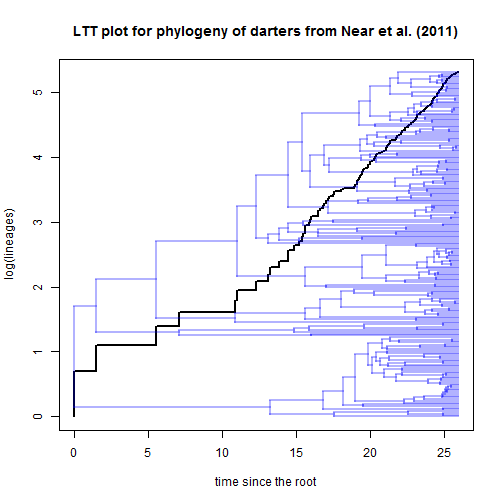## Tuesday, October 24, 2017

### More on fitting & comparing basic diversification models using phytools

I have now added a function to fit a Yule (pure-birth) model to phytools, as well as an S3 `logLik` method. This allows us to fit both a birth-death model & a pure-birth model, and then compare them in a relatively straightforward way.

Here's an example using a phylogeny of darters from Near et al. (2011).

``````library(phytools)
invisible(ltt(rotateNodes(darter.tree,"all"),show.tree=TRUE,lwd=2,
xlab="time since the root"))
title(main="LTT plot for phylogeny of darters from Near et al. (2011)")
``````Let's fit our models. According to the study, there are actually 216 lineages in this clade, although the tree contains only 201 of these. We can now take this into account.

``````Yule<-fit.yule(multi2di(darter.tree),sampling.f=Ntip(darter.tree)/216)
Yule
``````
``````##
## Fitted Yule model:
##
## ML(b/lambda) = 0.2289
## log(L) = 370.8404
##
## Assumed sampling fraction (rho) = 1
##
## R thinks it has converged.
``````
``````BD<-fit.bd(multi2di(darter.tree),sampling.f=Ntip(darter.tree)/216)
BD
``````
``````##
## Fitted birth-death model:
##
## ML(b/lambda) = 0.2362
## ML(d/mu) = 0.015
## log(L) = 370.8757
##
## Assumed sampling fraction (rho) = 1
##
## R thinks it has converged.
``````
``````library(lmtest)
lrtest(Yule,BD)
``````
``````## Likelihood ratio test
##
## Model 1: Yule
## Model 2: BD
##   #Df LogLik Df  Chisq Pr(>Chisq)
## 1   1 370.84
## 2   2 370.88  1 0.0706     0.7905
``````

This tells us, of course, that we cannot reject the simpler Yule model.

Similarly:

``````aic<-setNames(c(AIC(Yule),AIC(BD)),c("Yule","birth-death"))
aic.w(aic)
``````
``````##        Yule birth-death
##   0.7240612   0.2759388
``````

shows that the majority of weight of evidence favors the Yule model for this tree.

Neat.